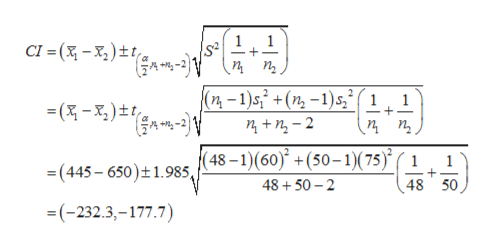# Researchers compared cortisol levels of two samples of women at childbirth. The first group (n=48) underwent emergency cesarean section following induced labor and had a mean cortisol level of 445 with a standard deviation of 60. The second group (n=50) delivered either by cesarean section or vaginally following spontaneous labor, and had a mean cortisol level of 650 with a standard deviation of 75. Construct a two-sided 95% confidence interval for the difference between the two means. Assume equal variances. Give an interpretation of the confidence interval. You MUST show your work to receive full credit. Partial credit is available.

Question
1 views

Researchers compared cortisol levels of two samples of women at childbirth. The first group (n=48) underwent emergency cesarean section following induced labor and had a mean cortisol level of 445 with a standard deviation of 60. The second group (n=50) delivered either by cesarean section or vaginally following spontaneous labor, and had a mean cortisol level of 650 with a standard deviation of 75. Construct a two-sided 95% confidence interval for the difference between the two means. Assume equal variances. Give an interpretation of the confidence interval. You MUST show your work to receive full credit. Partial credit is available.

check_circle

Step 1

Solution:

The critical value is obtained below:

From the given information, the first group sample size n1 is 48, standard deviation s1 is 60 and mean is 445. The second group sample size n2 is 50, standard deviation s1 is 75 and mean is 650. The confidence level is 95%.

The level of significance=1-0.95=0.05.

The degrees of freedom= n1+n2–2=48+50-2=96

The critical value is,

From the EXCEL, using the formula, =T.INV.2T(0.05,5), the critical value is 1.985.

Step 2

The two-sided 95% confidence interval for the difference between the two means is constructed below:

The required 95%confidence interval is,help_outlineImage TranscriptioncloseS& 1 п п, CI=(x-)tt (n-1)s +(n-1)s,1 1 nn-2 п, (48-1)(60) +(50-1)(75) 48+50 2 1 (445 650)t1.985, 48 50 -(-232.3,-177.7) fullscreen
Step 3

The confidence interval is interpreted below:

There is 95% confident that difference between the two means lies between &nda...

### Want to see the full answer?

See Solution

#### Want to see this answer and more?

Solutions are written by subject experts who are available 24/7. Questions are typically answered within 1 hour.*

See Solution
*Response times may vary by subject and question.
Tagged in

### Other